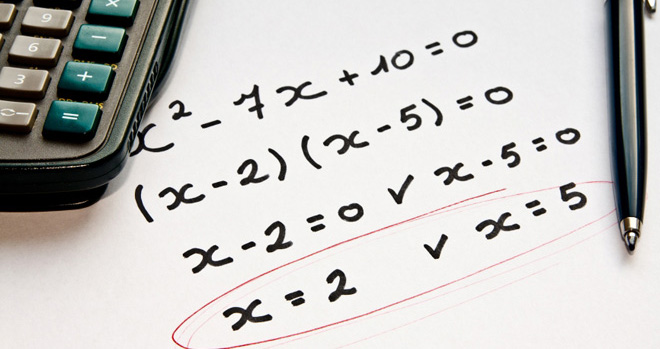Learn the 4 Things You Must Know Before Taking the GMAT!   Download it Now!

# Learn to Dominate GMAT AlgebraWe go to great lengths at Dominate the GMAT to help you avoid doing difficult algebra on the GMAT. There are useful non-standard math techniques, for example, that can help you make variables your friend and turn otherwise problematic word problems into run-of-the-mill math problems.

Sometimes, however, solving algebra problems on the GMAT is simply unavoidable.

In this course you will learn how to tackle several of the most commonly-tested “difficult” algebra concepts tested on the GMAT including quadratic equations, simultaneous equations, and inequalities. We’ll also cover GMAT algebra questions that involve powers (exponents) and roots (radicals) as well as algebraic expressions that you may need to either simplify or manipulate in some way. But don’t worry — there won’t be a lot of long formulas to memorize (we still remember a song our 7th grade algebra teacher taught us to help us remember the Quadratic Formula, and don’t worry, we won’t be signing it to you here!); rather, we’ll focus on teaching you how the GMAT tests each of these topics and equip you with plenty of examples to illustrate everything you’ll need to master these GMAT algebra problem types and get more right answers on test day.

Specifically, the GMAT video tutorials in this course cover:

• Simultaneous equations, with multiple approaches and strategies so that you can choose the method that works best for you;
• GMAT quadratic equations, with an emphasis on the three most commonly-tested quadratic equations on the GMAT;
• The FOIL Method, including how to factor quadratic equations using the “reverse FOIL” method;
• Figuring out how many roots a quadratic equation has for determining sufficiency on GMAT Data Sufficiency questions;
• The in’s and out’s of inequalities;
• GMAT exponents, including combining exponents by addition/subtraction and multiplication/division as well as a fool-proof strategy for avoiding the most common exponent traps found on the GMAT;
• Detailed review of roots and radicals;
• The most commonly-tested exponents and square roots on the GMAT;
• Simplifying algebraic expressions;
• Algebraic functions;
• Numerous application examples to fully illustrate all of the concepts taught in this course;
• And more!

Oh, and did we mention that this course includes a practice worksheet that will give you the opportunity to apply what you learn to real sample GMAT algebra questions? Each question of the worksheet comes with a detailed video answer explanation showing you the most efficient way to solve it.

So what are you waiting for? Take your GMAT preparation to the next level. Add this course to your library now and prepare to dominate the GMAT!

### Cost: Only \$27!